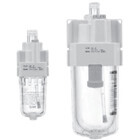AL10-A to AL60-A, Lubricator, Metric North American & European Product Update: !!For U.S. users, SMC recommends and encourages the use of a metal bowl or bowl guard for size "20" units!!

Configuration OptionsSelect Product Options
 Select values from the drop down lists or make appropriate selections to configure the part number. Body Size -- Pick an Option -- 10 20 30 40 50 60Thread -- Pick an Option -- Rc (Rc: for Sizes 20~60; M5: for Size 10) N (NPT) F (G)Port Size -- Pick an Option -- M5 (M5 x 0.8) 01 (1/8) 02 (1/4) 03 (3/8) 04 (1/2) 06 (3/4) 10 (1)Mounting -- Pick an Option -- None B (Bracket)Semi-Standard, Body Size 10 -- Pick an Option -- Standard Z (Name Plate, Caution Plate for Bowl in Imperial Units: psi, °F) 2 (Metal Bowl) 2R (2 + R) 2RZ (2 + R + Z) 2Z (2 + Z) 23 (2 + 3) 23R (2 + 3+ R) 23Z (2 + 3 + Z) 23RZ (2 + 3 + R + Z) 3 (Drain Cock) 3R (3 + R) 3RZ (3 + R + Z) 3Z (3 + Z) 36 (3 + 6) 36R (3 + 6 + R) 36RZ (3 + 6 + R + Z) 36Z (3 + 6 + Z) 6 (Nylon Bowl) 6R (6 + R) 6RZ (6 + R + Z) 6Z (6 + Z) R (Flow Direction: Right to Left) RZ (R + Z)Semi-Standard, Body Size 20 -- Pick an Option -- Standard Z (Name Plate, Caution Plate for Bowl in Imperial Units: psi, °F) 2 (Metal Bowl) 2Z (2 + Z) 2R (2 + R) 2RZ (2 + R + Z) 23 (2 + 3) 23R (2 + 3+ R) 23Z (2 + 3 + Z) 23RZ (2 + 3 + R + Z) 3 (Drain Cock) 3C (3 + C) 3CR (3 + C + R) 3CRZ (3 + C + R + Z) 3CZ (3 + C + Z) 3R (3 + R) 3RZ (3 + R + Z) 3Z (3 + Z) 36 (3 + 6) 36R (3 + 6 + R) 36Z (3 + 6 + Z) 36RZ (3 + 6 + R + Z) 6 (Nylon Bowl) 6C (w/Bowl Guard, Nylon Bowl) 6CR (6 + C + R) 6CRZ (6 + C + R + Z) 6R (6 + R) 6RZ (6 + R + Z) 6Z (6 + Z) C (Bowl Guard) CR (C + R) CRZ (C + R + Z) CZ (C + Z) R (Flow Direction: Right to Left) RZ (R + Z)Semi-Standard, Body Size 30, 40, 50 & 60 -- Pick an Option -- Standard Z (Name Plate, Caution Plate for Bowl in Imperial Units: psi, °F) 2 (Metal Bowl) 2R (2 + R) 2RZ (2 + R + Z) 2Z (2 + Z) 23 (2 + 3) 23R (2 + 3 + R) 23Z (2 + 3 + Z) 23RZ (2 + 3 + R + Z) 3 (Drain Cock) 3R (3 + R) 3RW (3 + R + W) 3RWZ (3 + R + W + Z) 3RZ (3 + R + Z) 3W (3 + W) 3WZ (3 + W + Z) 3Z (3 + Z) 36 (3 + 6) 36R (3 + 6 + R) 36Z (3 + 6 + Z) 36RZ (3 + 6 + R + Z) 38 (3 + 8) 38R (3 + 8 + R) 38RZ (3 + 8 + R + Z) 38Z (3 + 8 + Z) 6 (Nylon Bowl) 6R (6 + R) 6RZ (6 + R + Z) 6Z (6 + Z) 8 (Metal Bowl w/Level Gauge) 8R (8 + R) 8RZ (8 + R + Z) 8Z (8 + Z) R (Flow Direction: Right to Left) RZ (R + Z)Options in: Gray are not compatible with other selected option value. Red conflict with another selected option value. Amber are not recommended.

 FavoritesCustomer Part Number:
 Linear Actuators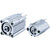C(D)Q2-Z Dbl Act,w/Auto Sw Mounting Groove Valves & Manifolds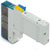SY3000, 5 Port Solenoid Valve, All Types - New Style Airline Equipment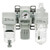AC20-B to AC60-B, Air Filter, Regulator and Lubricator Fittings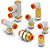One-touch Fitting, Inch Size Tube, UNF, NPT Connection Thread Tubing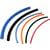Polyurethane, Metric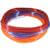Nylon, Inch